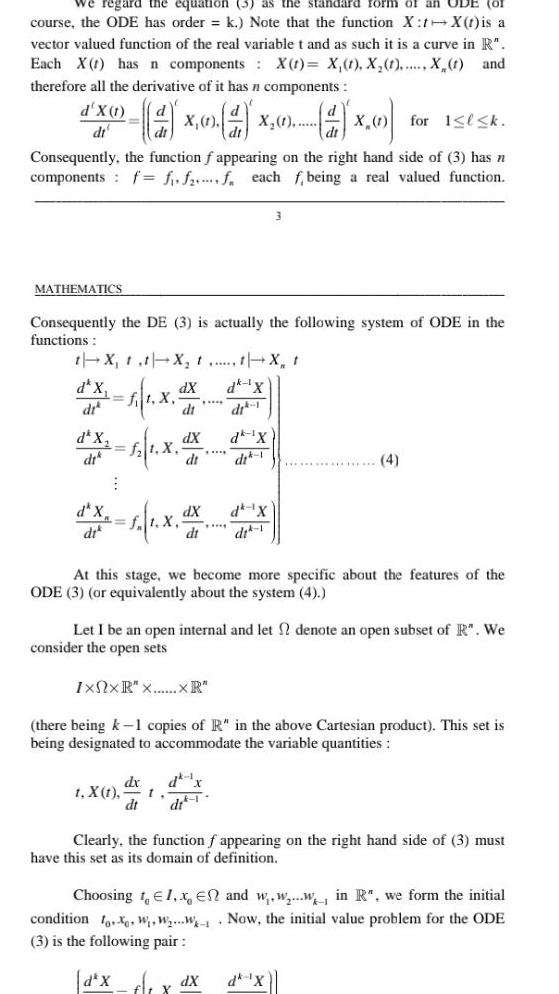Question:

# we regard the equation 3 as standard form of an UDE OI

Last updated: 9/18/2023we regard the equation 3 as standard form of an UDE OI course the ODE has order k Note that the function X tX t is a vector valued function of the real variable t and as such it is a curve in R Each X has n components X t X 1 X 1 X t and therefore all the derivative of it has n components X 1 X 1 for 1 k Consequently the function f appearing on the right hand side of 3 has n components f f ff each f being a real valued function d X 1 dt MATHEMATICS Consequently the DE 3 is actually the following system of ODE in the functions t X t t X t t X t d X dX 1 X d di d X dr 1 1 X d X di ft X t X t dX d X dt dit 1 dX dt dx dt 1 3 At this stage we become more specific about the features of the ODE 3 or equivalently about the system 4 Let I be an open internal and let denote an open subset of R We consider the open sets Ixnx R xXR there being k 1 copies of R in the above Cartesian product This set is being designated to accommodate the variable quantities 4 dit 1 Clearly the function f appearing on the right hand side of 3 must have this set as its domain of definition dX dk X Choosing 1 1 x En and www in R we form the initial Now the initial value problem for the ODE condition fo W WW 3 is the following pair d X f